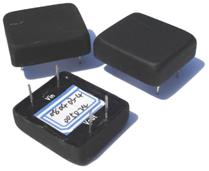# Step Down Radial Piezoelectric Transformers

#### Piezoelectric Transformers### T1-Transoner - 15W

Piezoelectric transformer designed for compact fluorescent lamps (approx. 500 to 1000 ohm load)
Size (mm): D=19.05 x t=3.81
Resonance Freq: 121 kHz ± 5%
Transfer ratio: 1±0.1 (RL=500 ohm)
Efficiency > 95% (at RL=500 ohm)### Step Down Piezo Transformer family rated at 5W

Size (mm): L=29 x W=25.5 x t=8.7
Resonance Freq:
Input voltage:
Power: 5W max
Step-up:
Efficiency: >### Step Down Piezo Transformer family rated at 10W

Size (mm): L=29 x W=25.5 x t=8.7
Resonance Freq:
Input voltage:
Power: 10W max
Step-up:
Efficiency: >### Step Down Piezo Transformer family rated at 20W

Size (mm): L=37 x W=33.5 x t=8.8
Resonance Freq:
Input voltage:
Power: 20W max
Step-up:
Efficiency: >### Step Down Piezo Transformer family rated at 30W

Size (mm): L=41 x W=37.5 x t=9
Resonance Freq:
Input voltage:
Power: 30W max
Step-up:
Efficiency: >

### Step Down Piezo Transformer family rated at 50W

Size (mm): L=46 x W=42.5 x t=9
Resonance Freq:
Input voltage:
Power: 50W max
Step-up:
Efficiency: >